header > paper > figures > footer

# Visualizing Dynamical Systems near Critical Points

by H. Löffelmann, H. Doleisch, and E. Gröller.

Project Duration: 1996 - 1998.

This page assembles some results (figures) of work that is part of our research topic ``Visualization of Complex Dynamical Systems''. The figures are provided in JPEG format.header < paper > figures > footerheader < paper < figures > footer

## Figures in the paper (JPEG)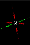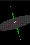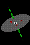Figure 1a, Figure 1b, Figure 1c: Visualizing the geometry of behavior near the critical point of a linear dynamical system - three different saddle configurations.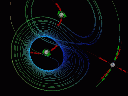Figure 2a: Visualizing the flow characteristics near the critical points of the Lorenz system - eigen manifold visualization.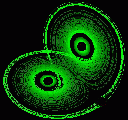Figure 2a: Visualizing the flow characteristics near the critical points of the Lorenz system - bunches of streamlets.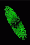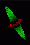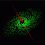Figure 3a, Figure 3b, Figure 3c: Visualizing a linear system near the critical point with a pair of conjugated complex eigen values: a repellor (a) and a saddle (b,c).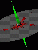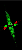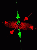Figure 4a, Figure 4b, Figure 4c: Three different saddle configurations visualized using both the eigen manifold visualization and bunches of streamlets.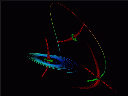Figure 5: Visualizing the flow near the critical points of the Lorenz system - both bunches of streamlets and eigen manifold visualization were used.header < paper < figures < more images < footer

Helwig Löffelmann, last update on March 10, 1998.